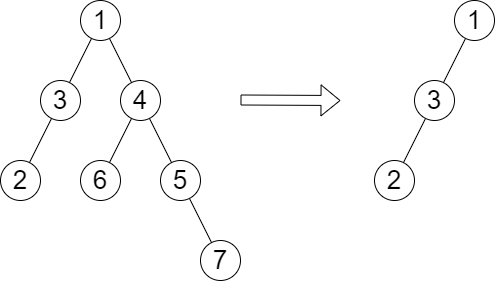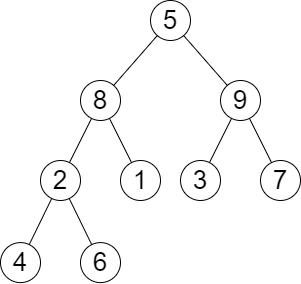##### Welcome to Subscribe On Youtube

Formatted question description: https://leetcode.ca/all/2458.html

# 2458. Height of Binary Tree After Subtree Removal Queries

• Difficulty: Hard.
• Related Topics: Array, Tree, Depth-First Search, Breadth-First Search, Binary Tree.
• Similar Questions: Maximum Depth of Binary Tree.

## Problem

You are given the root of a binary tree with n nodes. Each node is assigned a unique value from 1 to n. You are also given an array queries of size m.

You have to perform m independent queries on the tree where in the ith query you do the following:

• Remove the subtree rooted at the node with the value queries[i] from the tree. It is guaranteed that queries[i] will not be equal to the value of the root.

Return an array **answer of size m where answer[i] is the height of the tree after performing the ith query**.

Note:

• The queries are independent, so the tree returns to its initial state after each query.

• The height of a tree is the number of edges in the longest simple path from the root to some node in the tree.

Example 1:Input: root = [1,3,4,2,null,6,5,null,null,null,null,null,7], queries = 
Output: 
Explanation: The diagram above shows the tree after removing the subtree rooted at node with value 4.
The height of the tree is 2 (The path 1 -> 3 -> 2).


Example 2:Input: root = [5,8,9,2,1,3,7,4,6], queries = [3,2,4,8]
Output: [3,2,3,2]
Explanation: We have the following queries:
- Removing the subtree rooted at node with value 3. The height of the tree becomes 3 (The path 5 -> 8 -> 2 -> 4).
- Removing the subtree rooted at node with value 2. The height of the tree becomes 2 (The path 5 -> 8 -> 1).
- Removing the subtree rooted at node with value 4. The height of the tree becomes 3 (The path 5 -> 8 -> 2 -> 6).
- Removing the subtree rooted at node with value 8. The height of the tree becomes 2 (The path 5 -> 9 -> 3).


Constraints:

• The number of nodes in the tree is n.

• 2 <= n <= 105

• 1 <= Node.val <= n

• All the values in the tree are unique.

• m == queries.length

• 1 <= m <= min(n, 104)

• 1 <= queries[i] <= n

• queries[i] != root.val

## Solution (Java, C++, Python)

• /**
* Definition for a binary tree node.
* public class TreeNode {
*     int val;
*     TreeNode left;
*     TreeNode right;
*     TreeNode() {}
*     TreeNode(int val) { this.val = val; }
*     TreeNode(int val, TreeNode left, TreeNode right) {
*         this.val = val;
*         this.left = left;
*         this.right = right;
*     }
* }
*/
class Solution {
private Map<TreeNode, Integer> d = new HashMap<>();
private int[] res;

public int[] treeQueries(TreeNode root, int[] queries) {
f(root);
res = new int[d.size() + 1];
d.put(null, 0);
dfs(root, -1, 0);
int m = queries.length;
int[] ans = new int[m];
for (int i = 0; i < m; ++i) {
ans[i] = res[queries[i]];
}
return ans;
}

private void dfs(TreeNode root, int depth, int rest) {
if (root == null) {
return;
}
++depth;
res[root.val] = rest;
dfs(root.left, depth, Math.max(rest, depth + d.get(root.right)));
dfs(root.right, depth, Math.max(rest, depth + d.get(root.left)));
}

private int f(TreeNode root) {
if (root == null) {
return 0;
}
int l = f(root.left), r = f(root.right);
d.put(root, 1 + Math.max(l, r));
return d.get(root);
}
}

• /**
* Definition for a binary tree node.
* struct TreeNode {
*     int val;
*     TreeNode *left;
*     TreeNode *right;
*     TreeNode() : val(0), left(nullptr), right(nullptr) {}
*     TreeNode(int x) : val(x), left(nullptr), right(nullptr) {}
*     TreeNode(int x, TreeNode *left, TreeNode *right) : val(x), left(left), right(right) {}
* };
*/
class Solution {
public:
vector<int> treeQueries(TreeNode* root, vector<int>& queries) {
unordered_map<TreeNode*, int> d;
function<int(TreeNode*)> f = [&](TreeNode* root) -> int {
if (!root) return 0;
int l = f(root->left), r = f(root->right);
d[root] = 1 + max(l, r);
return d[root];
};
f(root);
vector<int> res(d.size() + 1);
function<void(TreeNode*, int, int)> dfs = [&](TreeNode* root, int depth, int rest) {
if (!root) return;
++depth;
res[root->val] = rest;
dfs(root->left, depth, max(rest, depth + d[root->right]));
dfs(root->right, depth, max(rest, depth + d[root->left]));
};
dfs(root, -1, 0);
vector<int> ans;
for (int v : queries) ans.emplace_back(res[v]);
return ans;
}
};

• # Definition for a binary tree node.
# class TreeNode:
#     def __init__(self, val=0, left=None, right=None):
#         self.val = val
#         self.left = left
#         self.right = right
class Solution:
def treeQueries(self, root: Optional[TreeNode], queries: List[int]) -> List[int]:
def f(root):
if root is None:
return 0
l, r = f(root.left), f(root.right)
d[root] = 1 + max(l, r)
return d[root]

def dfs(root, depth, rest):
if root is None:
return
depth += 1
res[root.val] = rest
dfs(root.left, depth, max(rest, depth + d[root.right]))
dfs(root.right, depth, max(rest, depth + d[root.left]))

d = defaultdict(int)
f(root)
res =  * (len(d) + 1)
dfs(root, -1, 0)
return [res[v] for v in queries]


• /**
* Definition for a binary tree node.
* type TreeNode struct {
*     Val int
*     Left *TreeNode
*     Right *TreeNode
* }
*/
func treeQueries(root *TreeNode, queries []int) (ans []int) {
d := map[*TreeNode]int{}
var f func(*TreeNode) int
f = func(root *TreeNode) int {
if root == nil {
return 0
}
l, r := f(root.Left), f(root.Right)
d[root] = 1 + max(l, r)
return d[root]
}
f(root)
res := make([]int, len(d)+1)
var dfs func(*TreeNode, int, int)
dfs = func(root *TreeNode, depth, rest int) {
if root == nil {
return
}
depth++
res[root.Val] = rest
dfs(root.Left, depth, max(rest, depth+d[root.Right]))
dfs(root.Right, depth, max(rest, depth+d[root.Left]))
}
dfs(root, -1, 0)
for _, v := range queries {
ans = append(ans, res[v])
}
return
}

func max(a, b int) int {
if a > b {
return a
}
return b
}


Explain:

nope.

Complexity:

• Time complexity : O(n).
• Space complexity : O(n).